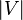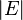# Bellman-Ford algorithm facts for kids

Kids Encyclopedia Facts

In computer science, Bellman-Ford is an algorithm used to compute the shortest distances and paths from a single node within a graph to all other nodes.

## Usage

It is more robust than Dijkstra's algorithm because it is able to handle graphs containing negative edge weights. It may be improved by noting that, if an iteration of the main loop of the algorithm completes without making any changes, the algorithm can be immediately completed, as future iterations will not make any changes. Its runtime is$O(|V|\cdot |E|)$ time, where$|V|$ and$|E|$ are the number of nodes and edges respectively.

## Psuedocode

function BellmanFord(list nodes, list edges, node source) is



distance := list predecessor := list // Predecessor holds shortest paths

    // Step 1: initialize
for each node n in nodes do


distance[n] := inf // Initialize the distance to all nodes to infinity predecessor[n] := null // And having a null predecessor




distance[source] := 0 // The distance from the source to itself is zero

    // Step 2: relax edges repeatedly
repeat |nodes|−1 times:
for each edge (u, v) with weight w in edges do
if distance[u] + w < distance[v] then


distance[v] := distance[u] + w predecessor[v] := u

    // Step 3: check for negative-weight cycles
for each edge (u, v) with weight w in edges do
if distance[u] + w < distance[v] then
error "Graph contains a negative-weight cycle"

return distance, predecessorBellman-Ford algorithm Facts for Kids. Kiddle Encyclopedia.